# Logarithmic mapping allows for low discount factors by creating action gaps similar in size

This post has been republished via RSS; it originally appeared at: Microsoft Research.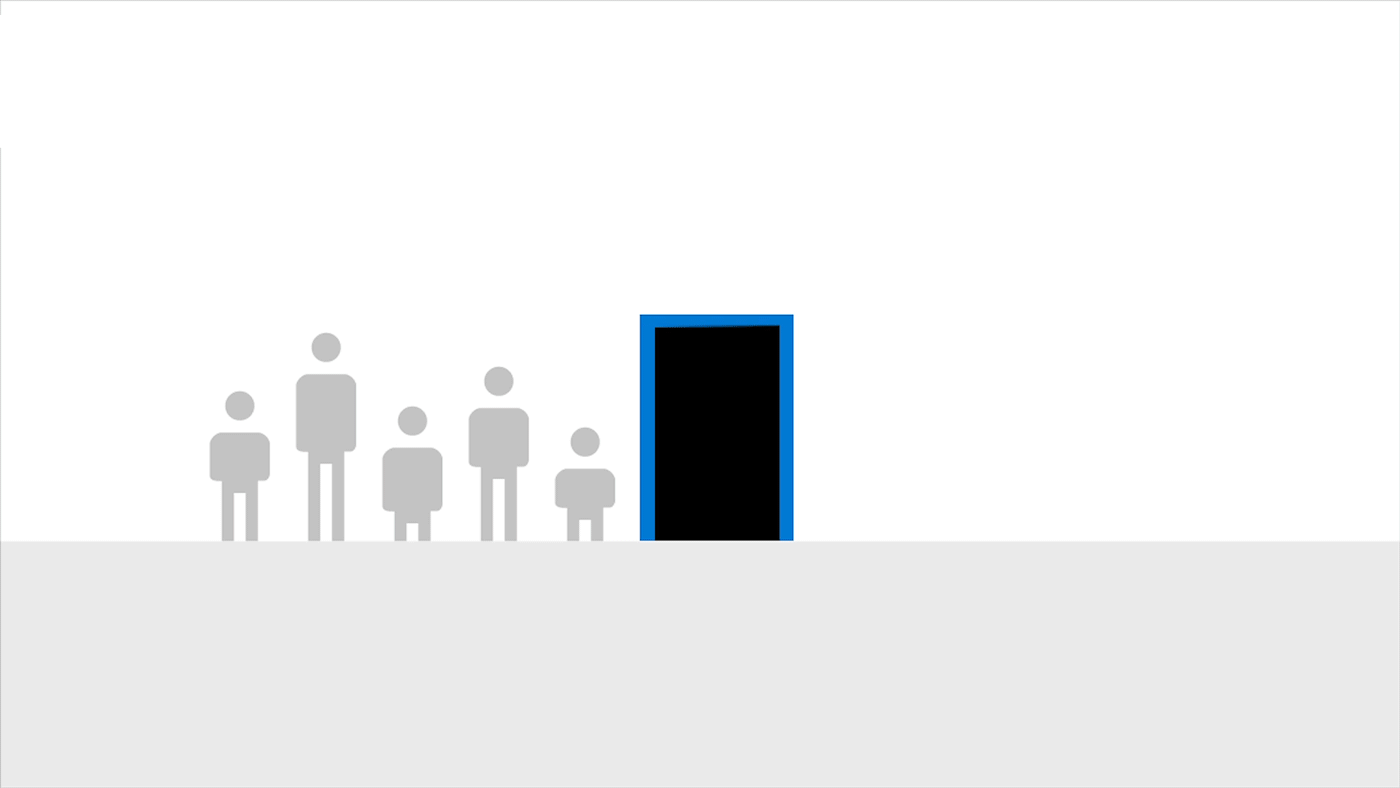While reinforcement learning (RL) has seen significant successes over the past few years, modern deep RL methods are often criticized for how sensitive they are with respect to their hyper-parameters. One such hyper-parameter is the discount factor, which controls how future rewards are weighted compared to immediate rewards.

The objective that one wants to optimize in RL is often best described as an undiscounted sum of rewards (for example, maximizing the total score in a game). In practice, however, a discount factor is introduced to avoid some of the optimization challenges that can occur when directly optimizing on an undiscounted objective. And while in theory a discount factor can take on any value between 0 and 1, in reality good performance is only obtained for a small subset of values close to 1.

In our paper to be presented at the thirty-third Conference on Neural Information Processing Systems (NeurIPS 2019), called “Using a Logarithmic Mapping to Enable Lower Discount Factors in Reinforcement Learning,” we present a novel hypothesis as to why the effective discount-factor range is so small. Also, based on our hypothesis, we outline a technique to avoid this discount-factor sensitivity and introduce a method, which we call logarithmic Q-learning, based on this technique. Logarithmic Q-learning is the first method able to achieve good performance for low discount factors on sparse-reward tasks with function approximation. In addition, the method not only reduces discount-factor sensitivity but can also improve performance altogether.

### With standard reinforcement learning methods, low discount factors don’t work

On sparse-reward tasks with function approximation, performance drops dramatically if the discount factor is too low. We illustrate this by considering a chain task (see Figure 1) consisting of a sequence of states with two actions: left and right. The left action aims to move the agent one state to the left, while the right action aims to move the agent to the right. Due to environmental stochasticity, however, an action only results in its intended behavior with a probability of 75% and results in a move in the opposite direction with a probability of 25%. All actions result in a reward of 0, except when the left or right terminal state is reached, which results in a reward of +1 or -1 respectively.

It is easy to see that the optimal policy for this task is to always take the left action—regardless of the discount factor. However, when we try to learn this policy by optimizing for the discounted sum of rewards using a linear representation based on tile coding, the learning behavior shows a very strong dependence on the discount factor (see Figure 1). In particular, if the discount factor value falls below some threshold, a standard RL method is not able to learn the optimal policy.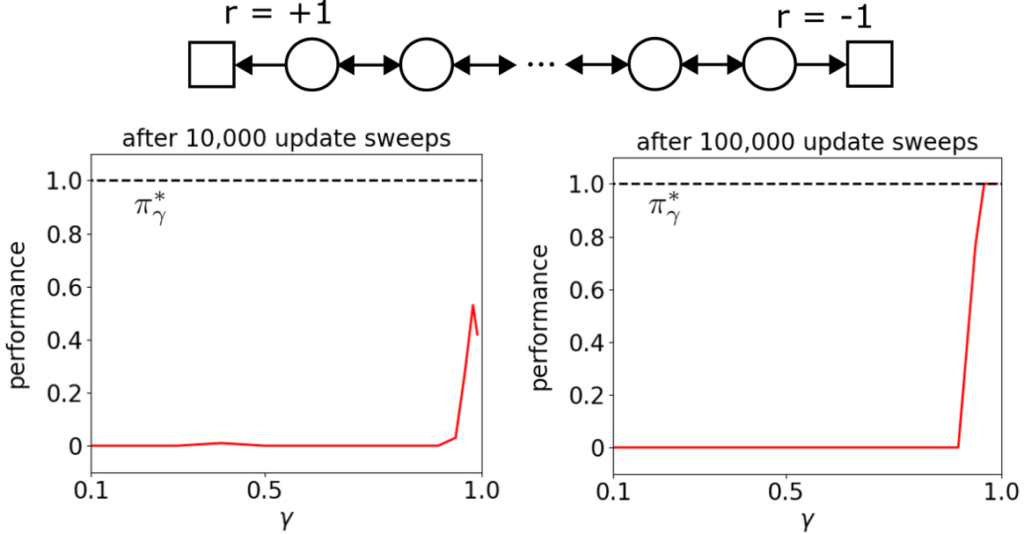Figure 1: Performance of a standard RL method after training on the chain task shown at the top, as well as the performance of the optimal policy πγ*. For low discount factors, performance drops dramatically.

To try to understand the performance drop, we turn to the optimal Q-value function, which gives the expected, discounted sum of rewards under the optimal policy for each state-action combination. For small discount factors, the Q-value difference between an optimal and a non-optimal action of a state, called the action gap, becomes very small. So, a common explanation for the poor performance of low discount factors is these small action gaps because it is believed that these are detrimental to the optimization process when function-approximation errors are present.

Surprisingly, after empirically testing this hypothesis, this turned out not to be the cause (while there is correlation, there is no causation; see the paper for details). We came up with an alternative hypothesis, which states that a large size difference of the action gap across the state space is the main culprit. If this hypothesis is correct, it means that if a strategy were found to make the action gap more homogenous in size across the state space, performance for small discount factors should go up.

### The solution strategy: Using a logarithmic mapping function

The action gaps across the state space can be made more homogenous by mapping the Q-value function to a logarithmic space. We illustrate this below using a deterministic variation of the chain task where the left terminal state results in +1 reward, while the right terminal state results in a reward of +0.5.

On this task, the difference between the optimal Q-value of the left action and the optimal Q-value of the right action corresponds with the size of the action gap. Additionally, a positive difference means the left action is optimal, while a negative difference means the right action is optimal. In Figure 2, the Q-value difference is shown across the state space for different values of the discount factor; the graph on the right (labeled “after”) shows the difference after applying a logarithmic mapping to the Q-value function.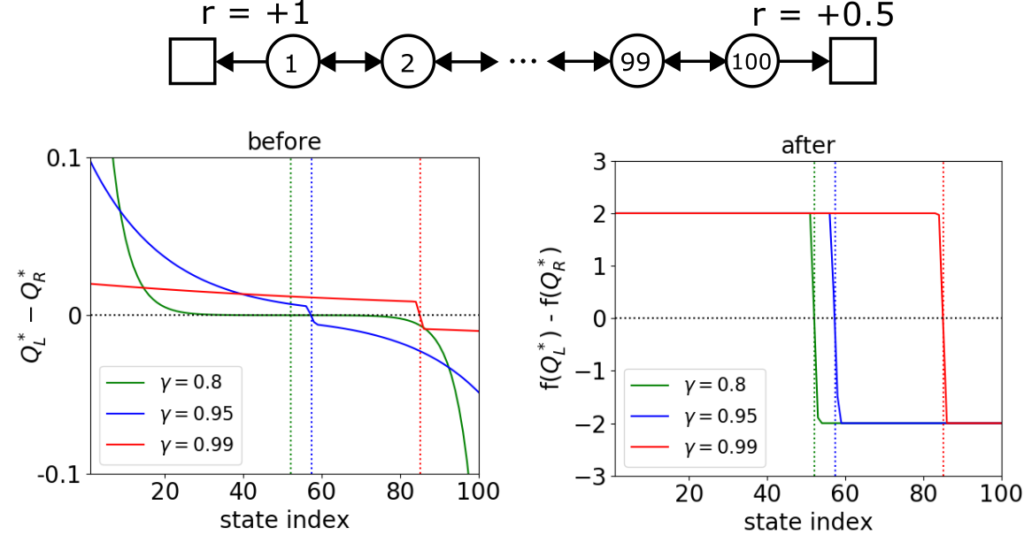Figure 2: Effect of a logarithmic mapping on the action gap. After applying the logarithmic mapping, the action gap becomes similar in size across the state space. The vertical dotted lines indicate the state where the Q-value difference changes sign, corresponding with a change of optimal action.

While a logarithmic mapping function makes action gaps more homogenous, there are a number of challenges to overcome to build a robust algorithm, based on this outcome, that can be applied to stochastic domains with both positive and negative rewards. We managed to overcome these challenges and develop an algorithm, logarithmic Q-learning, that can be applied to general tasks and has convergence guarantees under standard conditions. Figure 3 shows the performance of this algorithm on the initial chain task. These results do not only show great performance for low discount factors. Early performance of high discount factors is also better than it was without the logarithmic mapping.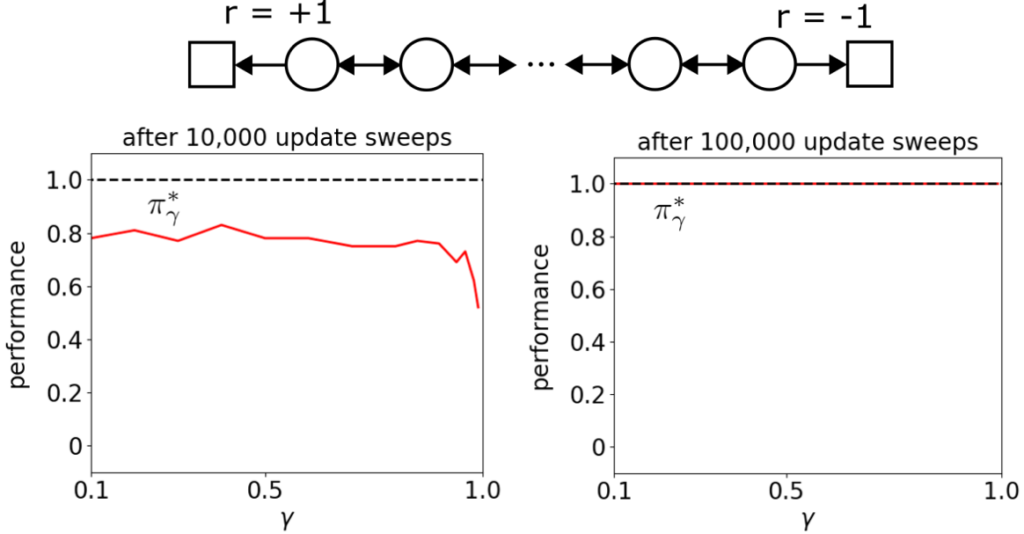Figure 3: Performance of logarithmic Q-learning after training. Note that after 100,000 update sweeps, the learned policy has converged to the optimal policy πγ* for all discount factors. Compared to the results without logarithmic mapping (Figure 1), the robustness with respect to the discount factor has improved dramatically.

### Results on Atari games: Performance of DQN when logarithmic mapping is added

To study the effect on larger domains, we developed a variation of DQN that uses a logarithmic mapping, which we call LogDQN. In Figure 4, the performance of LogDQN compared to DQN is plotted for 55 Atari games, while Figure 5 shows the mean and median human-normalized performance. Overall, these results show that using a logarithmic mapping can also be beneficial for larger domains.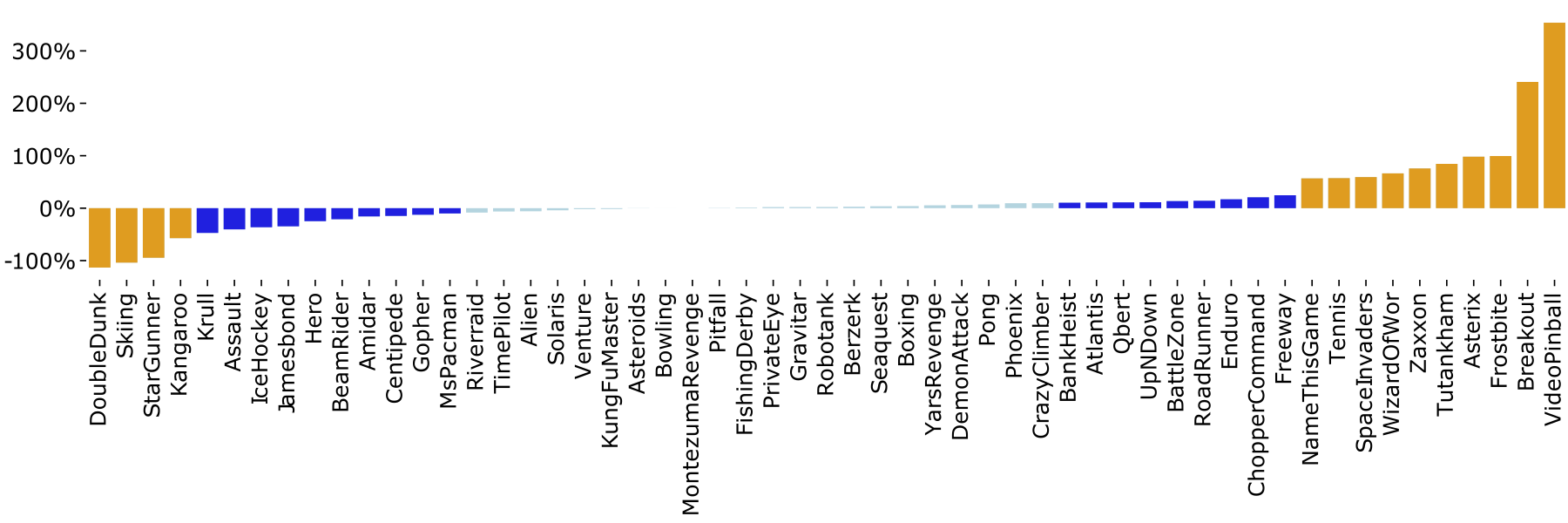Figure 4: Relative performance of LogDQN when compared with DQN. A positive percentage means LogDQN outperforms DQN; a negative percentage means DQN outperforms LogDQN.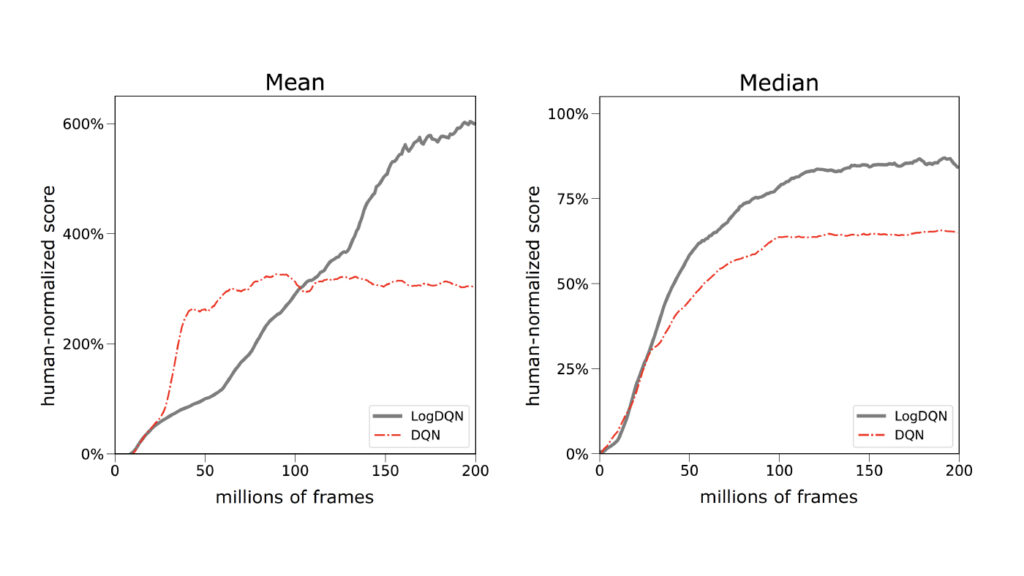Figure 5: Mean and median human-normalized performance during training across 55 Atari games. A human-normalized score of 0% means the performance is the same as that of a random policy; a score of 100% corresponds with human performance.

Our results highlight the importance of having action gaps that are homogenous in size across the state space. While small discount factors can induce action gaps that are orders of magnitude different in size across the state space, using a logarithmic mapping function is a natural remedy against this. There are many different types of logarithmic mapping functions. The one we used in this work is especially effective for sparse-reward domains. An interesting future research direction would be to explore other logarithmic mapping functions as well as other effects the discount factor has on optimization.

This work has been accepted for oral presentation at NeurIPS 2019. It will be presented at 4:50pm PST during Track 3 Session 2. We encourage you to test the results for yourself and explore the details of the code used for our experiments, which can be found here: https://github.com/microsoft/logrl

This site uses Akismet to reduce spam. Learn how your comment data is processed.